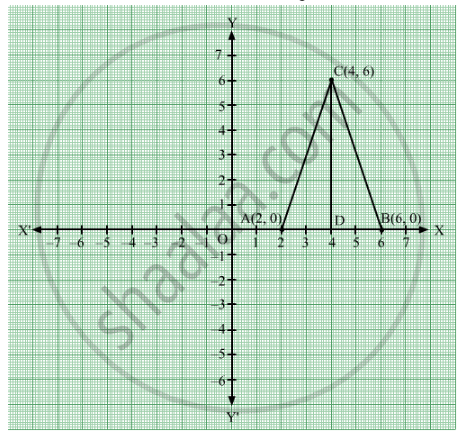# The Area of the Triangle Formed by the Points A(2,0) B(6,0) and C(4,6) is - Mathematics

MCQ

The area of the triangle formed by the points A(2,0) B(6,0)  and C(4,6) is

#### Options

•  24 sq. units

• 12 sq. units

• 10 sq. units

• none of these

#### Solution

Given that points A(2,0), B(6,0) and C(4 ,6) form a triangle which is shown in the figure. We are asked to find the area of the triangle ΔABC.Given that

OA = 2

and OB = 6

Hence

$\text { AB = OB - OA}$

$= 6 - 2$

$= 4$

CD = 6

By using formula,

$∆\text { ABC }= \frac{1}{2} \times \text { AB} \times \text{CD}$

$= \frac{1}{2} \times 4 \times 6$

$= 12 \text { sq units}$

Concept: Coordinate Geometry
Is there an error in this question or solution?

#### APPEARS IN

RD Sharma Mathematics for Class 9
Chapter 8 Co-ordinate Geometry
Exercise 8.2 | Q 14 | Page 7

Share